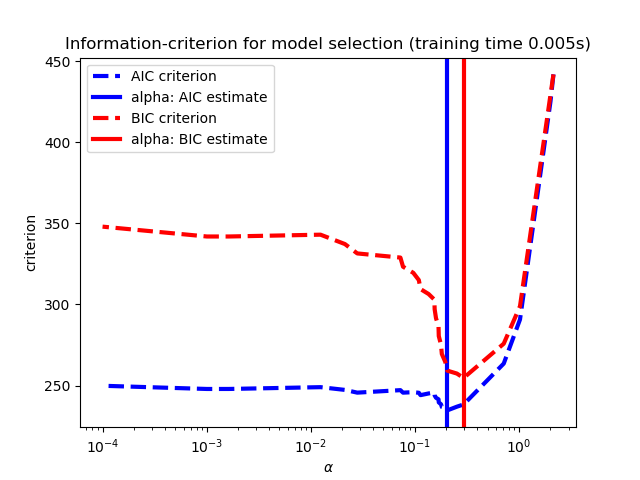# sklearn.linear_model.LassoLarsIC¶

class sklearn.linear_model.LassoLarsIC(criterion='aic', *, fit_intercept=True, verbose=False, normalize=True, precompute='auto', max_iter=500, eps=2.220446049250313e-16, copy_X=True, positive=False)

Lasso的优化目标是：

AIC是Akaike信息标准，而BIC是Bayes信息标准。通过在拟合优度和模型复杂度之间进行权衡，这样的标准对于选择正则化参数的值很有用。一个好的模型应该在简单的同时很好地解释数据。

criterion {‘bic’ , ‘aic’}, default=’aic’

fit_intercept bool, default=True

verbose bool or int, default=False

normalize bool, default=False
fit_intercept设置为False 时，将忽略此参数。如果为True，则在回归之前通过减去均值并除以l2-范数来对回归变量X进行归一化。如果你希望标准化，请先使用 sklearn.preprocessing.StandardScaler，然后调用fit 估算器并设置normalize=False
precompute bool, ‘auto’ or array-like , default=’auto’

max_iter int, default=500

eps float, optional
Cholesky对角因子计算中的机器精度正则化。对于病态系统，请增加此值。与某些基于迭代优化的算法中的tol参数不同，此参数不控制优化的容忍度。默认情况下，使用np.finfo(np.float).eps
copy_X bool, default=True

positive bool, default=False

coef_ array-like of shape (n_features,)

intercept_ float

alpha_ float

n_iter_ int
lars_path运行以查找alpha网格的迭代次数。
criteria_like array-like of shape (n_alphas,)

“On the degrees of freedom of the lasso” Hui Zou, Trevor Hastie, and Robert Tibshirani Ann. Statist. Volume 35, Number 5 (2007), 2173-2192.

https://en.wikipedia.org/wiki/Akaike_information_criterion https://en.wikipedia.org/wiki/Bayesian_information_criterion

>>> from sklearn import linear_model>>> reg = linear_model.LassoLarsIC(criterion='bic')>>> reg.fit([[-1, 1], [0, 0], [1, 1]], [-1.1111, 0, -1.1111])LassoLarsIC(criterion='bic')>>> print(reg.coef_)[ 0.  -1.11...]

fit(X, y[, copy_X]) 使用X，y作为训练数据拟合模型。
get_params([deep]) 获取此估计量的参数。
predict(X) 使用线性模型进行预测。
score(X, y[, sample_weight]) 返回预测的确定系数R ^ 2。
set_params(**params) 设置此估算器的参数。
__init__（standard ='aic'，*，fit_intercept = True，verbose = False，normalize = True，precompute ='auto'，max_iter = 500，eps = 2.220446049250313e-16，copy_X = True，positive = False ）

[源码]

fit(X, y, copy_X=None)

[源码]

X array-like of shape (n_samples, n_features)

y array-like of shape (n_samples,)

copy_X bool, default=None

self object

get_params(deep=True)

[源码]

deep bool, default=True

params mapping of string to any

predict(X)

[源码]

X array_like or sparse matrix, shape (n_samples, n_features)

C array, shape (n_samples,)

score(X, y, sample_weight=None)

[源码]

X array-like of shape (n_samples, n_features)

y array-like of shape (n_samples,) or (n_samples, n_outputs)
X的真实值。
sample_weight array-like of shape (n_samples,), default=None

score float

set_params(**params)

[源码]

**params dict

self object

## sklearn.linear_model.LassoLarsIC使用示例¶